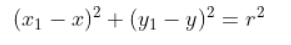# How to get random coordinates from inside a circle present on canvas

I want to get random coordinates from circle
For example
If coordinate (x,y) is acting like a centre of circle with radius K
I want random coordinates present inside the circle.

Even a small idea would be great
Thank you

See the example below I have a circle with center (0,0) and r = 4. Use the following equationwhere x,y are coordinates of center , x1,y1 are coordinates of point and r = radius
In the following example
B = (0,4) so from the equation you get 16=16 so point is on circle,
D = (-2,1) so from the equation you get 5 < 16 so point is inside circle
E = (-6,2) so from the equation you get 40 > 16 so point is outside circle

Maybe this might help you to check if random coordinates created by a procedure are inside circle or not

1 Like

There is error in formula still I got bit idea

I think I will have to generate a random number for x<r then use that as range for y.

I will keep you updated.

1 Like

Formula corrected. Working with Latex sometimes leads to errors1 Like

This topic was automatically closed 30 days after the last reply. New replies are no longer allowed.